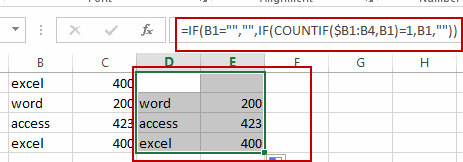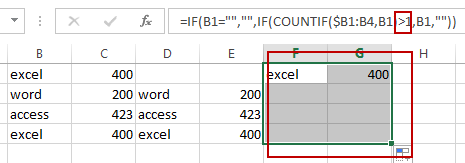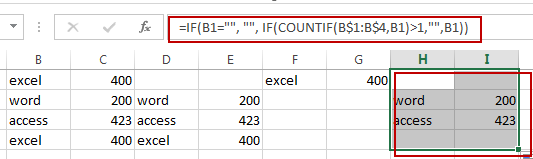# How to Replace Duplicates with Blank Cells in Excel

This post will guide you how to remove duplicates and replace with blank cells in Excel 2013/2016. How do I replace the duplicates with blank cells using a formula in Excel.

## Replace Duplicates with Blank Cells

Assuming that you have a list of data in range B1:C4, and you want to remove duplicate values in this range of cells and replace it with blank cells. How to achieve it.  And if you just use the Remove Duplicates command, it will remove all duplicate rows only. So you can use a formula to remove duplicates and replace it with blank cell based on the IF function, and the COUNTIF function.

Just like this:

`=IF(B1="","",IF(COUNTIF(\$B1:B4,B1)=1,B1,""))`

Type this formula into a blank cell, such as: D1 and press Enter key in your keyboard, and then drag the AutoFill Handle down to Cell F4 to apply this formula.## Show Only Duplicate Values

If you want to replace all unique values with blank cells and only show duplicates values in Excel, you can use another formula based on the IF function and COUNTIF function. Like this:

`=IF(B1="","",IF(COUNTIF(\$B1:B4,B1)>1,B1,""))`

Type this formula into a blank cell and then drag the AutoFill Handle down to other cells to apply this formula.

Let’s see the result:## Show Only Unique Values

If you want only to show the unique values and remove all duplicate values and replace with blank cells in Excel, you can use the following formula based on the IF and COUNTIF function.

`=IF(B1="", "", IF(COUNTIF(B\$1:B\$4,B1)>1,"",B1))`Video: Replace Duplicates with Blank Cells

### Related Functions

• Excel IF function
The Excel IF function perform a logical test to return one value if the condition is TRUE and return another value if the condition is FALSE.The syntax of the IF function is as below:= IF (condition, [true_value], [false_value])…
• Excel COUNTIF function
The Excel COUNTIF function will count the number of cells in a range that meet a given criteria. This function can be used to count the different kinds of cells with number, date, text values, blank, non-blanks, or containing specific characters.etc.= COUNTIF (range, criteria)…
Related Posts

Phone Number Format in Excel

Sometimes when we import phone numbers from external sources, the formatting of the phone numbers is removed. Even if in some situations the phone numbers are not imported from external resource, sometimes the phone numbers in worksheet are not displayed ...

If Cell Contain Specific Text

This article will explain how to check if a cell contains text or a specific text string in Google Sheets or Microsoft Excel spreadsheets. In our daily work, we often look for specific text in worksheets containing large amounts of ...

Cell Contains Number

This article will talk about how to check if a given cell contains any numbers in Microsoft Excel Spreadsheet or Google Sheets. How to quickly check if a cell contains a number? When you have a small number of cells ...

Calculate Days Open in Excel

If you want to know how to Calculate days in Excel, there are some formulas that you can use to do so. For example, you can use the DAYS function in Excel to find the number of days between two ...

Add Row Numbers And Skip Blanks in Excel

Do you ever have to input a list of numbers into a spreadsheet, and some of the cells are blank? It can be difficult to keep track of what number is in which cell when you have to scroll up ...

Sidebar